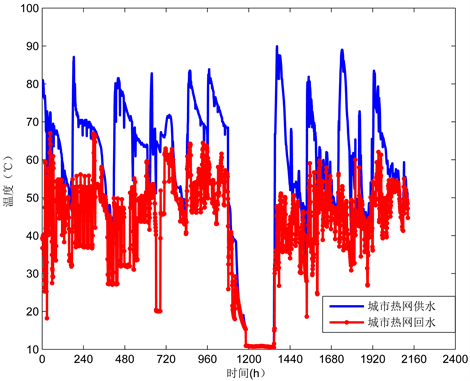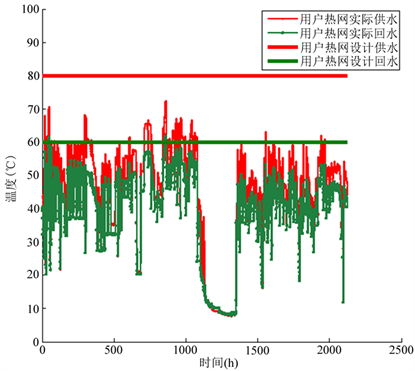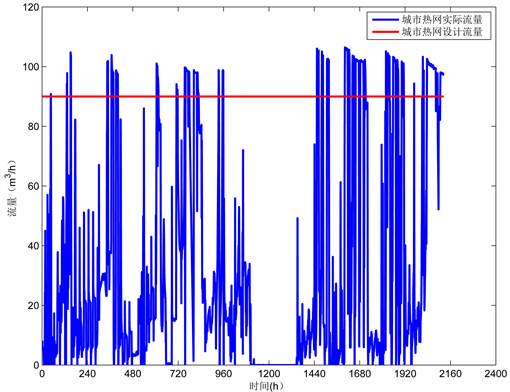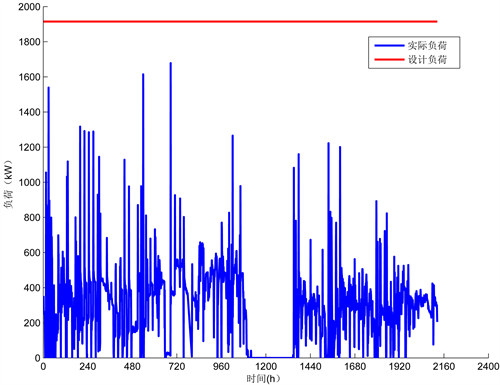# 基于精细化控制的供热系统节能分析Energy Saving Analysis of Heat Supply System Based on Elaborate Control

DOI: 10.12677/HJCE.2018.75080, PDF, HTML, XML, 下载: 693  浏览: 911  科研立项经费支持

Abstract: Comprehensively considering various factors such as weather conditions, building properties, users’ habits and so on, the heat load of the district heating system is predicted in real time by using the neural network algorithm. To eliminate the effect of building thermal inertia, as well as the time delay in heat supply system and heat transfer process, the distributed frequency conversion technology is used to actively and continuously adjust the operating mode of the primary pumps in the heat source plant and each heat consuming installation. Based on data wireless transmission technology, the linkage control of heating station and heat exchange station is realized. The elaborate control strategy has achieved excellent operating state and energy saving effect when applied to a district heating system in a heat supply company in Xi’an. The energy saving rate is above 50%, which indicates great application value.

1. 引言

2. 理论耗热量

2.1. 住宅建筑

$q=\frac{20-2.6}{18-\left(-3.4\right)}×40=32.52$ W/m2

$Q=32.52×120×24×3600/{10}^{9}=0.3372$ GJ/m2

2.2. 办公建筑

$q=\left[\frac{20-2.6}{18-\left(-3.4\right)}×\frac{15}{24}+\frac{5-2.6}{18-\left(-3.4\right)}×\frac{9}{24}\right]×60=33.01$ W/m2

$Q=33.01×120×24×3600/{10}^{9}=0.3422$ GJ/m2

$Q=60×120×24×3600/{10}^{9}=0.6221$ GJ/m2

2.3. 宾馆建筑

$q=\frac{22-2.6}{18-\left(-3.4\right)}×45=40.79$ W/m2

$Q=40.79×120×24×3600/{10}^{9}=0.4229$ GJ/m2

2.4. 工业建筑

$q=\left[\frac{20-2.6}{18-\left(-3.4\right)}×\frac{15}{24}+\frac{5-2.6}{18-\left(-3.4\right)}×\frac{9}{24}\right]×50=27.51$ W/m2

$Q=27.51×120×24×3600/{10}^{9}=0.2852$ GJ/m2

3. 集中供热系统精细化控制

3.1. 分布式变频系统设计

3.1.1. 分布式变频系统原理

3.1.2. 分布式变频系统设计

1) 管网系统进行水力计算，确定系统所需的流量和压力分布。

2) 按照供热的经济性，合理选择压差控制点位置，一般控制在热源厂内部。

3) 选择主循环泵，流量满足全网流量需求，扬程克服压差控制点之前的系统阻力。

4) 选择用户一次水泵，满足该分支用户的阻力和流量。用户一次水泵应满足用户近、远期负荷发展要求，并能适应外网压力变化。

3.2. 集中供热系统精细化控制

3.2.1. 负荷预测

3.2.2. 检测参数

3.2.3. 控制对象

3.2.4. 控制方法

${t}_{pj}=\frac{{t}_{g}+{t}_{h}}{2}=\frac{{q}_{h}}{{q}_{h0}}\left({t}_{pj0}-{t}_{n0}\right)+{t}_{n}$ (1)

$\frac{\left({\tau }_{1}-{t}_{g}\right)-\left({\tau }_{2}-{t}_{h}\right)}{\mathrm{lg}\frac{{\tau }_{1}-{t}_{g}}{{\tau }_{2}-{t}_{h}}}=\frac{\left({\tau }_{10}-{t}_{g0}\right)-\left({\tau }_{20}-{t}_{h0}\right)}{\mathrm{lg}\frac{{\tau }_{10}-{t}_{g0}}{{\tau }_{20}-{t}_{h0}}}\frac{{q}_{h}}{{q}_{h0}}$ (2)

4. 运行效果分析

4.1. 设计条件

2016~2017年采暖季共有4家用户投入运行，分别为第一学校、第六小学、公安局、消防站。各换热站设计条件见表1 (其中，第一学校换热站夜间仅维持5℃的值班温度)。

4.2. 运行效果

2016~2017年采暖季的实际运行效果表明，基于精细化控制的供热系统具有十分可观的节能潜力。对于夜间仅维持值班温度的热用户(第一学校)，节能潜力尤为可观(表2)。表中所有用户的实际耗热量均小于理论耗热量，平均实际耗热量指标为0.1569 GJ/m2，平均实际热负荷仅15.13 W/m2Table 1. Design conditions of heating users in 2016-2017Table 2. Operating results of heating users in 2016-2017Figure 1. Curve: supply and return temperature of urban heating networkFigure 2. Curve: supply and return temperature of user’s heating networkFigure 3. Curve: flow rate of urban heating network5. 结论与建议

  彭琛, 江亿. 中国建筑节能路线图[M]. 北京: 中国建筑工业出版社, 2015: 76-79.  江亿. 中国建筑及节能理念思辨[M]. 北京: 中国建筑工业出版社, 2016: 87-91.  中华人民共和国住房和城乡建设部. CJJ 34-2010城镇供热管网设计规范[S]. 北京: 中国建筑工业出版社, 2010.